# a simple pendulum of frequency f has a metal bob.If the bob us negatively charged and is allowed to oscillate with a posively charged plate under it , the frequency will..???

10 years ago

A pendulum is a weight suspended from a pivot so that it can swing freely. When a pendulum is displaced sideways from its resting equilibrium position, it is subject to a restoring force due to gravity that will accelerate it back toward the equilibrium position. When released, the restoring force combined with the pendulum''s mass causes it to oscillate about the equilibrium position, swinging back and forth. The time for one complete cycle, a left swing and a right swing, is called the period. A pendulum swings with a specific period which depends (mainly) on its length. From its discovery around 1602 by Galileo Galilei the regular motion of pendulums was used for timekeeping, and was the world''s most accurate timekeeping technology until the 1930s. Pendulums are used to regulate pendulum clocks, and are used in scientific instruments such as accelerometers and seismometers. Historically they were used as gravimeters to measure the acceleration of gravity in geophysical surveys, and even as a standard of length. The word ''pendulum'' is new Latin, from the Latin pendulus, meaning ''hanging''. The simple gravity pendulum is an idealized mathematical model of a pendulum. This is a weight (or bob) on the end of a massless cord suspended from a pivot, without friction. When given an initial push, it will swing back and forth at a constant amplitude. Real pendulums are subject to friction and air drag, so the amplitude of their swings declines.

10 years ago

# Period of Simple Pendulum

A point mass hanging on a massless string is an idealized example of a simple pendulum. When displaced from its equilibrium point, the restoring force which brings it back to the center is given by:For small angles θ, we can use the approximation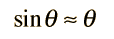in which case Newton''s 2nd law takes the form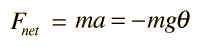Even in this approximate case, the solution of the equation uses calculus and differential equations. The differential equation is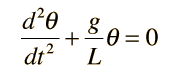and for small angles θ the solution is: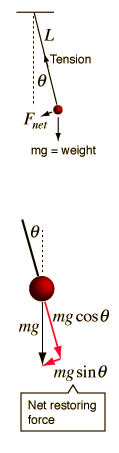Index

Periodic motion concepts

 R Nave
Go Back

# Pendulum Geometry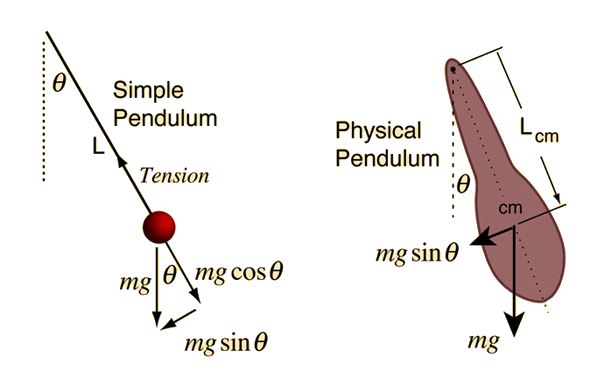HyperPhysics***** Mechanics R Nave
Go Back

# Pendulum Equation

The equation of motion for the simple pendulum for sufficiently small amplitude has the form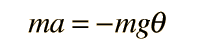which when put in angular form becomesThis differential equation is like that for the simple harmonic oscillator and has the solution: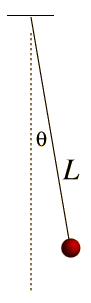The motion of a simple pendulum is like simple harmonic motion in that the equation for the angular displacement is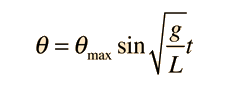which is the same form as the motion of a mass on a spring:The anglular frequency of the motion is then given by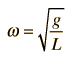compared tofor a mass on a spring.The frequency of the pendulum in Hz is given by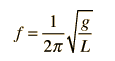and the period of motion is then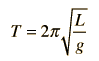10 years ago

The frequency will probably decrease.

10 years ago

DUE TO ATTRACTION THERE WILL BE TWO ACCLERATION ONE ACCELERATION DUE TO GRAVITY AND ACCELERATION DUE TO ATTRACTION THEREFORE ''G'' EFFECTIVE WILL DECREASE HENCE TIME PERIOD WILL INCREASE AND HENCE FREQUENCY WILL DECREASE PLZZZZZZZZZ APPROVE